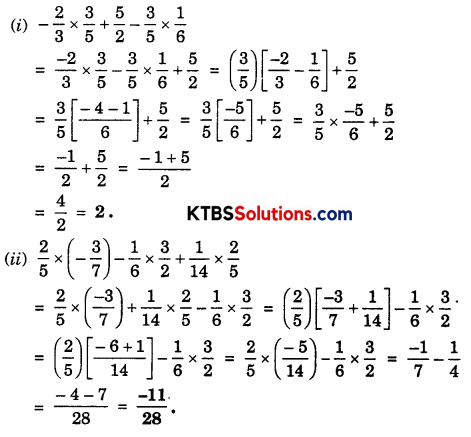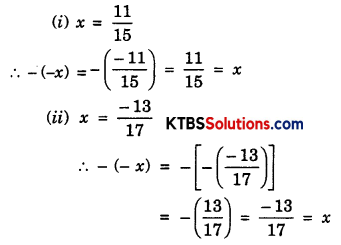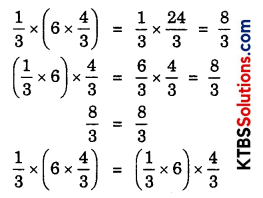# KSEEB Solutions for Class 8 Maths Chapter 1 Rational Numbers Ex 1.1

## KSEEB Solutions for Class 8 Maths Chapter 1 Rational Numbers Ex 1.1

Question 1.
Using appropriate properties find:
(i) $$-\frac{2}{3} \times \frac{3}{5}+\frac{5}{2}-\frac{3}{5} \times \frac{1}{6}$$
(ii) $$\frac{2}{5} \times\left(-\frac{3}{7}\right)-\frac{1}{6} \times \frac{3}{2}+\frac{1}{14} \times \frac{2}{5}$$
Solution:Question 2.
Write the additive inverse of each of the following:
(i) $$\frac{2}{8}$$
(ii) $$\frac{-5}{9}$$
(iii) $$\frac{-6}{-5}$$
(iv) $$\frac{2}{-9}$$
(v) $$\frac{19}{-6}$$
Solution:
(i) The additive inverse of $$\frac{2}{8}$$ is $$\frac{-2}{8}$$.

(ii) The additive inverse of $$\frac{-5}{9}$$ is $$-\left(\frac{-5}{9}\right)$$ = $$\frac{5}{9}$$

(iii) $$\frac{-6}{-5}=\frac{6}{5}$$
∴ The additive inverse of $$\frac{6}{5}$$ is $$\frac{-6}{5}$$.

(iv) $$\frac{2}{-9}=\frac{-2}{9}$$
∴ The additive inverse of $$\frac{-2}{9}$$ is $$\frac{2}{9}$$.

(v) $$\frac{19}{-6}=\frac{-19}{6}$$
∴ The additive inverse of $$\frac{-19}{6}$$ is $$\frac{19}{6}$$.Question 3.
Verify that -(-x) = x for:
(i) x = $$\frac{11}{15}$$
(ii) x = $$-\frac{13}{17}$$
Solution:Question 4.
Find the multiplicative inverse of the following:
(i) -13
(ii) $$\frac{-13}{19}$$
(iii) $$\frac{1}{5}$$
(iv) $$\frac{-5}{8} \times \frac{-3}{7}$$
(v) $$-1 \times \frac{-2}{5}$$
(vi) -1
Solution:
(i) The multiplicative inverse of -13 is $$\frac{1}{-13}$$.

(ii) The multiplicative inverse of $$\frac{-13}{19}$$ is $$\frac{1}{\frac{-13}{19}}=\frac{19}{-13}$$.

(iii) The multiplicative inverse of $$\frac{1}{5}$$ is 5.

(iv) The multiplicative inverse of $$\frac{-5}{8} \times \frac{-3}{7}=\frac{15}{56}$$ is $$\frac{56}{15}$$.

(v) The multiplicative inverse of $$-1 \times \frac{-2}{5}=\frac{2}{5}$$ is $$\frac{5}{2}$$.

(vi) The multiplicative inverse of -1 is $$\frac{1}{-1}$$ = -1.

Question 5.
Name the property under multiplication used in each of the following:
(i) $$\frac{-4}{5} \times 1=1 \times \frac{-4}{5}=-\frac{4}{5}$$
(ii) $$-\frac{13}{17} \times \frac{-2}{7}=\frac{-2}{7} \times \frac{-13}{17}$$
(iii) $$\frac{-19}{29} \times \frac{29}{-19}=1$$
Solution:
(i) Multiplicative inverse.
(ii) Commutative.
(iii) 1, is the multiplicative identity.Question 6.
Multiply $$\frac{6}{13}$$ by the reciprocal of $$\frac{-7}{16}$$
Solution:
The reciprocal of $$\frac{-7}{16}$$ is $$\frac{16}{-7}=\frac{6}{13} \times \frac{16}{-7}=\frac{96}{-91}$$

Question 7.
Tell what property allows you to compute $$\frac{1}{3} \times\left(6 \times \frac{4}{3}\right)$$ as $$\left(\frac{1}{3} \times 6\right) \times \frac{4}{3}$$
Solution:For any three rational numbers a, b and c
a × (b × c) = (a × b) × c
The multiplication is associative for rational numbers.

Question 8.
Is $$\frac{8}{9}$$ the multiplicative inverse of $$-1 \frac{1}{8}$$? Why or why not?
Solution:
Multiplicative inverse of $$\frac{8}{9}$$ is $$\frac{9}{8}$$ or $$1 \frac{1}{8}$$
But, here $$-1 \frac{1}{8}$$ is negative.
∴ $$\frac{8}{9}$$ is not multiplicative inverse of $$-1 \frac{1}{8}$$.

Question 9.
Is 0.3 the multiplicative inverse of $$3 \frac{1}{3}$$? Why or why not?
Solution:
0.3 = $$\frac{3}{10}$$
Multiplicative inverse of $$\frac{3}{10}$$ is $$\frac{10}{3}$$ = $$3 \frac{1}{3}$$
Hence, 0.3 is multiplicative inverse of $$3 \frac{1}{3}$$
Since $$\frac{3}{10} \times \frac{10}{3}=1$$
If product of a number and its multiplicative inverse is 1.Question 10.
Write:
(i) The rational number that does not have a reciprocal.
(ii) The rational numbers that are equal to their reciprocals.
(iii) The rational number that is equal to its negative.
Solution:
(i) Zero is the rational number, that does not have reciprocal.
(ii) 1 is such a rational number, which is equal to its reciprocal. (-1) is also such a rational number.
(iii) 0 is a number which is equal to its negative.

Question 11.
Fill in the blanks:
(i) Zero has ________ reciprocal.
(ii) The numbers _________ and ________ are their own reciprocals.
(iii) The reciprocal of -5 is ________
(iv) Reciprocal of $$\frac{1}{x}$$, where x ≠ 0 is ________
(v) The product of two rational numbers is always a ________
(vi) The reciprocal of a positive rational number is ________
Solution:
(i) No
(ii) 1, -1
(iii) $$\frac{-1}{5}$$
(iv) x
(v) Rational number
(vi) Positive